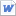검색어 입력폼

# 분자량을 측정하는방법 (GPC) 영어레포트

저작시기 2014.12 | 등록일 2014.12.29MS 워드 (docx) | 5페이지 | 가격 1,000원

## 소개글

분자량을 측정하는방법 (GPC) 영어레포트 입니다.

## 목차

1. Purpose of the experiment
2. Principle
3. Procedure
4. Data
5. Analysis
6. Conclusion

## 본문내용

Purpose of the experiment
Chromatography is skill for separating mixed matter. We got three unconfirmed samples. We want to know how many kinds of polymers are in the sample and molecular weight of each polymer.

Principle
GPC
Gel permeation chromatography (GPC) is a type of size exclusion chromatography (SEC), that separates analytes on the basis of size. The technique is often used for the analysis of polymers. We can investigate whole molar mass distribution.

PDI(Polydispersity Index)
In physical and organic chemistry, the dispersity is a measure of the heterogeneity of sizes of molecules or particles in a mixture. PDI is represented by the symbol Đ and calculated using the equation Đ = Mm/Mn, where Mm is the mass-average molar mass and Mn is the number-average molar mass

Definitions of molecular weight of polymer
Because polymer does not have specific molar mass, we can calculate the molar mass in several way. There are the number average molecular weight

없음